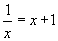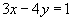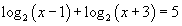equation

Equation

A mathematical sentence built from expressions using one or more equal signs (=).

Examples: